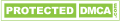# Industrial Terminology Introduction of Flow Meter

Flow metering is one of the most important parts in metering scientific technology, which has a close connection with national economy、national defense construction and scientific research. Flow metering is widely applied in varies of fields including industrial and agricultural production、national defense construction 、scientific research and foreign trade as well as people’s live, etc.
There is steam flow meter、revolving flow meter、electromagnetic flow meter and so on. More and more kinds of flow meters appearing in the market, resulting in the higher and higher requirements in model selection. In flow meter’s industry, here are some common used terms. I will mainly do some introduction about these terms as an editor of Side flow meter in Shanxi.

### 1.Flow range

Flow range of flow meter means that the measuring error can not surpass maximum and minimum range of allowable value in regular service conditions. The difference between maximum and minimum values is called the flow range. The ratio between maximum and minimum values is named the range ratio.

### 2.Rated flow

When flow meter has been set at a specified performance, a flow value will occur which is  called rated flow

### 3.Flow coefficient

The flow coefficient shows the ratio between actual flow and theoretical flow. Generally speaking, its influencing factors are rather complicated that it can not be gained by theoretical analysis. Instead, it can be made sure by experiment.

### 4.Instrument coefficient

The instrument coefficient is to via flow meter’s unit volume to get the corresponding signal pulsing number. It is a crucial parameter of signal pulse to output typed flow meter. At the same time, it is also a basis of this type about a mutual contact between once tested component and display instrument.

### 5.Characteristics curve of flow meter

Characteristics curve of flow meter is the ones that reflect the performance changes according to variation in discharge. Characteristics curve is usually used in two different representations: one is to show some characteristics of the flow meter( usually it mirrors discharge coefficient or instrument coefficient, it also mirrors the output concerning the flow), which has a relation with flow q or Reynolds number Re. The other is to demonstrate a relation between the measuring error of flow meter and the variation of flow q or Reynolds number Re. This characteristics curve is generally called the error characteristics curve of the flow meter.

Characteristics curve can be got through the theoretical analysis about flow meter. What’s more, there will be a more accurate one if testing the flow meter. That is, it can be gained by a series of real pulling within the whole flow range of flow meter.

### 6.Repeatability

The repeatability of flow meter is the meaning that this flow meter possesses an ability to give the same outcome when measuring one flow repeatedly and continuously. But attention should be paid that do not mix up the repeatability and accuracy. Accuracy means the competence of measuring a value most closed to the real value. If the repeatability of a flow meter is not good, there is no accuracy. But the excellent repeatability may have a same but not precise measurement result.

### 7.Linearity

The linearity of flow meter means the measurement about a degree of the characteristics curve deviating to fit a straight line. Sometimes it represents with a nonlinear error.
Related Articles